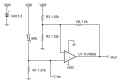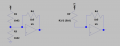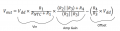# Temperature Sensing with NTC Circuit

#### andrew74

Joined Jul 25, 2022
102
I am reading this paper in which a circuit is explained to amplify with the voltage sensed by the change in resistance of an NTC.
There are some points that I do not understand that I would like to ask you:1) It is not clear to me how the Vout was found ... can you explain the reported formula?
..in particular, when developing the calculations (which I tried to do), I don't know where the -(R4/R3)*Vdd appears from.

2) When it says "calculate the value of R1 to produce a linear output voltage. Use the minimum and maximum values of the NTC to obtain a range of values for R1" ..
How did you find Rntc(max) and Rntc(min) ?
In theory, Rntc=R0*e^b(1/T-1/T0) ... but if I don't know what NTC was used, I don't know how much R0 and beta "b" are ... so I can't derive "Rntc". In my opinion, the choice is arbitrary and, therefore, this calculation should be done according to the NTC you have. Am I wrong?

3) What is Vin for? To correct any offset?

Last edited:

#### LowQCab

Joined Nov 6, 2012
3,451
Do You actually have the NTC-Resistor that You would like to use ?
Or is this just a "figure-figure" curiosity question ?

This particular Circuit arrangement is not one where You can figure-out all the
component values and expect it to be accurate.

Please let us know the purpose for this project, and all the various details,
if there is a use that You have in mind.
.
.
.

#### joeyd999

Joined Jun 6, 2011
4,971

#### andrew74

Joined Jul 25, 2022
102
If you have an MCU, op-amps are not required and only add to the error.
I read your post and I think it is better to place the NTC between the power supply and another resistor (and not the other way around where the NTC is grounded) because you can get an output voltage about linear with increasing temperature.

But, beyond this detail, I take the opportunity to ask what difference do you notice between a simple resistive divider (as you suggested) and the circuit with the opamp that to my inexperienced eyes apparently "looks" more accurate.
Do You actually have the NTC-Resistor that You would like to use ?
Or is this just a "figure-figure" curiosity question ?

This particular Circuit arrangement is not one where You can figure-out all the
component values and expect it to be accurate.

Please let us know the purpose for this project, and all the various details,
if there is a use that You have in mind.
.
.
.
For now I do not have to implement them, so the questions for an educational purpose remain valid

#### joeyd999

Joined Jun 6, 2011
4,971
I think it is better to place the NTC between the power supply and another resistor (and not the other way around where the NTC is grounded).
Having one terminal of a two terminal device grounded is better for a host of reasons -- in almost all circumstances. Noise, most importantly, especially if the NTC is remote -- which is usually the case.

...what difference do you notice between a simple resistive divider (as you suggested) and the circuit with the opamp...

I avoid superfluous components like the plague.

This approach has served me well over many decades.

#### andrew74

Joined Jul 25, 2022
102
Having one terminal of a two terminal device grounded is better for a host of reasons
Interesting!
Since I am new to this, if you have any sites or papers that explain these things better (I would not discuss it here because it is not the purpose of this thread) please attach them to me, you would be doing me a favor!
Thanks anyway#### joeyd999

Joined Jun 6, 2011
4,971
Interesting!
Since I am new to this, if you have any sites or papers that explain these things better (I would not discuss it here because it is not the purpose of this thread) please attach them to me, you would be doing me a favor!
Thanks anywayPapers?!

I'm a school-of-hard-knocks kinda guy.

•andrew74

#### Papabravo

Joined Feb 24, 2006
20,379
Interesting!
Since I am new to this, if you have any sites or papers that explain these things better (I would not discuss it here because it is not the purpose of this thread) please attach them to me, you would be doing me a favor!
Thanks anywayPapers? We're are hobbyists, not academics who can afford to let other people pay for access to the stuff behind the paywalls.

•Mullins

#### joeyd999

Joined Jun 6, 2011
4,971
We're are hobbyists...
Speak for yourself.

But I still don't do papers.

#### Papabravo

Joined Feb 24, 2006
20,379
Speak for yourself.

But I still don't do papers.
I thought I was, since most of us here are hobbyists. I have not read a paper in my entire professional career. I did have access to papers as a student, but I never paid much attention to them. even though they were free via the university library.

•joeyd999

#### joeyd999

Joined Jun 6, 2011
4,971

#### Irving

Joined Jan 30, 2016
3,496
Papers? We're are hobbyists, not academics
But I still don't do papers.
Come on guys, give the guy a break & get off your high horses, its a TI application note FFS; are you seriously saying you've never looked at an application note in your careers???1) It is not clear to me how the Vout was found ... can you explain the reported formula?
..in particular, when developing the calculations (which I tried to do), I don't know where the -(R4/R3)*Vdd appears from.
The opamp is configured as a differential amplifier, where both the inverting and non-inverting configuration are in use together. We can use Thevenin to simplify the R2||R3 by noting that R2=R3 so R2||R3 = 0.5R3 = R3' and the effective input voltage to the inverting side of the opamp is Vdd/2, eg:Now we can apply the superposition rule, and for each input set the other input to zero and calculate the output voltage.
For the NTC branch, its a non-inverting opamp:

$Vout(+) = Vdd * R1/(R_{NTC}+R1) * (1 + R4/R3') = Vdd * R1/(R_{NTC}+R1) * (1+2R4/R3)$

and for the other, its an inverting opamp:

$Vout(-) = -Vdd/2 * R4/R3' = -Vdd * R4/R3$

Combining...

$Vout = Vdd * (R1/(R_{NTC}+R1) * (1+2R4/R3) - R4/R3)$

The author of the app note used the identity R2||R3 = 0.5R3 in the -ve side, but chose to keep it as R2||R3 on the +ve side. This actually wrong, as the identity only works if R2 = R3, he should have used it both sides as I have, and stated the non-inverting gain in terms of R3 only by asserting R2 = R3.

#### joeyd999

Joined Jun 6, 2011
4,971
you've never looked at an application note in your careers?
I stopped looking because I hate errors.

•Irving

#### LowQCab

Joined Nov 6, 2012
3,451
Please let us know the purpose for this project, and all the various details,
if there is a use that You have in mind.
.
.
,

#### Irving

Joined Jan 30, 2016
3,496
I stopped looking because I hate errors.
Touche#### dcbingaman

Joined Jun 30, 2021
951
I am reading this paper in which a circuit is explained to amplify with the voltage sensed by the change in resistance of an NTC.
There are some points that I do not understand that I would like to ask you:

View attachment 292233

1) It is not clear to me how the Vout was found ... can you explain the reported formula?
..in particular, when developing the calculations (which I tried to do), I don't know where the -(R4/R3)*Vdd appears from.

2) When it says "calculate the value of R1 to produce a linear output voltage. Use the minimum and maximum values of the NTC to obtain a range of values for R1" ..
How did you find Rntc(max) and Rntc(min) ?
In theory, Rntc=R0*e^b(1/T-1/T0) ... but if I don't know what NTC was used, I don't know how much R0 and beta "b" are ... so I can't derive "Rntc". In my opinion, the choice is arbitrary and, therefore, this calculation should be done according to the NTC you have. Am I wrong?

3) What is Vin for? To correct any offset?
The reported formula can be broken down into the following parts:Vin -
The voltage at the non-inverting input. Is just a voltage divider resulting in a portion of Vdd applied to the amplifier.

Amp Gain -
Notice the amp is a non-inverting amplifier when you apply superposition thus Vdd on R3 is grounded, placing R3 and R2 in parallel. Thus the gain is just 1+(R4/(R2||R3)) which expressed using a common denominator is as shown.

Offset -
For the offset, ground 'Vin' and you get an inverting amplifier. I would normally say that is -(1/2)*Vdd*R4/(R3||R2). But because R3=R2 I can just replace R2 and R3 with say R and express it as -(1/2)*Vdd*R4/(R||R). Two resistors of the same value in parallel is the same as one resistor with half the value of R thus this is also:

-(1/2)*Vdd*R4/((1/2)*R)

But 1/2 is in both the numerator and denominator so it cancels leaving:

-Vdd*R4/R

But R3=R2=R so we can say it this way as well:

-Vdd*R4/R3 or -Vdd*R4/R2

That should answer the first question.

Last edited:
•andrew74 and Irving

#### Irving

Joined Jan 30, 2016
3,496
That should answer the first question.
As I did in post #12, but a second opinion never hurts...

#### dcbingaman

Joined Jun 30, 2021
951
I am reading this paper in which a circuit is explained to amplify with the voltage sensed by the change in resistance of an NTC.
There are some points that I do not understand that I would like to ask you:

View attachment 292233

1) It is not clear to me how the Vout was found ... can you explain the reported formula?
..in particular, when developing the calculations (which I tried to do), I don't know where the -(R4/R3)*Vdd appears from.

2) When it says "calculate the value of R1 to produce a linear output voltage. Use the minimum and maximum values of the NTC to obtain a range of values for R1" ..
How did you find Rntc(max) and Rntc(min) ?
In theory, Rntc=R0*e^b(1/T-1/T0) ... but if I don't know what NTC was used, I don't know how much R0 and beta "b" are ... so I can't derive "Rntc". In my opinion, the choice is arbitrary and, therefore, this calculation should be done according to the NTC you have. Am I wrong?

3) What is Vin for? To correct any offset?
The answer to the other two questions:

Rntc(max) and min. I think those can from some datasheet for a specific NTC. Though like you said, the document does not specify which one.

Vin. I think that is just trying to help explain the circuit so the document can talk about the voltage at the non-inverting input without needing to say that and instead just referring to is as Vin.

#### dcbingaman

Joined Jun 30, 2021
951
As I did in post #12, but a second opinion never hurts...
Exactly, I liked how you pointed out that part of the equation assumes R3 and R2 are not necessarily the same and another part assumes they are making the formula confusing and slightly misleading. I marked as like your #12 explanation.#### Irving

Joined Jan 30, 2016
3,496
In my opinion, the choice is arbitrary and, therefore, this calculation should be done according to the NTC you have. Am I wrong?
No, you are correct. Having said that a 2.25k @ 25C/815 @ 50C thermistor is a standard 'off the shelf' device, and would suit a wide range of situations, but I suspect was chosen for this app note as an 'easy' option.

•andrew74 and dcbingaman